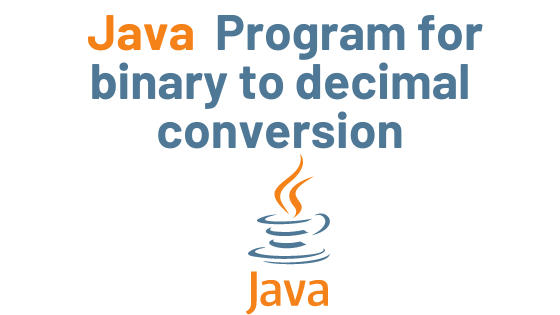## Java program for binary to decimal conversion with Example

There are two methods for converting a binary number to a decimal number(binary to decimal conversion):

1) Using the Integer class’s Integer.parseInt() method.
2) Perform conversion by writing your own logic rather than relying on predefined methods.

## Example: Binary to Decimal conversion using Integer.parseInt() method

```import java.util.Scanner;
class BinaryToDecimal {
public static void main(String args[]){
Scanner input = new Scanner( System.in );
System.out.print("Enter a binary number: ");
String binaryString =input.nextLine();
System.out.println("Output: "+Integer.parseInt(binaryString,2));
}
}```

Output:

Enter a binary number: 1101
Output: 13

## Example 2: Conversion without using parseInt

```public class Details {

public int BinaryToDecimal(int binaryNumber){

int decimal = 0;
int p = 0;
while(true){
if(binaryNumber == 0){
break;
} else {
int temp = binaryNumber%10;
decimal += temp*Math.pow(2, p);
binaryNumber = binaryNumber/10;
p++;
}
}
return decimal;
}

public static void main(String args[]){
Details obj = new Details();
System.out.println("110 --> "+obj.BinaryToDecimal(110));
System.out.println("1101 --> "+obj.BinaryToDecimal(1101));
System.out.println("100 --> "+obj.BinaryToDecimal(100));
System.out.println("110111 --> "+obj.BinaryToDecimal(110111));
}
}```

Fibonacci sequence Fibonacci sequence v Fibonacci sequence v Fibonacci sequence v Fibonacci sequence vFibonacci sequence

Output:

110 –> 6
1101 –> 13
100 –> 4
110111 –> 55

theudaipurstore.com

Java Basic Syntax | Java Tutorial

binary to decimal conversion binary to decimal conversion binary to decimal conversion binary to decimal conversion binary to decimal conversion binary to decimal conversion

In Java, encapsulation is a mechanism for combining variables (data) and methods (code) into a single unit. It is the process of concealing information details and safeguarding the object’s data and behavior. It’s one of the four key concepts in OOP. Because the encapsulating class is simple to test, it’s ideal for unit testing.In Java, encapsulation is a mechanism for combining variables (data) and methods (code) into a single unit. It is the process of concealing information details and safeguarding the object’s data and behavior. It’s one of the four key concepts in OOP. Because the encapsulating class is simple to test, it’s ideal for unit testing.In Java, encapsulation is a mechanism for combining variables (data) and methods (code) into a single unit. It is the process of concealing information details and safeguarding the object’s data and behavior. It’s one of the four key concepts in OOP. Because the encapsulating class is simple to test, it’s ideal for unit testing.In Java, encapsulation is a mechanism for combining variables (data) and methods (code) into a single unit. It is the process of concealing information details and safeguarding the object’s data and behavior. It’s one of the four key concepts in OOP. Because the encapsulating class is simple to test, it’s ideal for unit testing.In Java, encapsulation is a mechanism for combining variables (data) and methods (code) into a single unit. It is the process of concealing information details and safeguarding the object’s data and behavior. It’s one of the four key concepts in OOP. Because the encapsulating class is simple to test, it’s ideal for unit testing.In Java, encapsulation is a mechanism for combining variables (data) and methods (code) into a single unit. It is the process of concealing information details and safeguarding the object’s data and behavior. It’s one of the four key concepts in OOP. Because the encapsulating class is simple to test, it’s ideal for unit testing.In Java, encapsulation is a mechanism for combining variables (data) and methods (code) into a single unit. It is the process of concealing information details and safeguarding the object’s data and behavior. It’s one of the four key concepts in OOP. Because the encapsulating class is simple to test, it’s ideal for unit testing.In Java, encapsulation is a mechanism for combining variables (data) and methods (code) into a single unit. It is the process of concealing information details and safeguarding the object’s data and behavior. It’s one of the four key concepts in OOP. Because the encapsulating class is simple to test, it’s ideal for unit testing.In Java, encapsulation is a mechanism for combining variables (data) and methods (code) into a single unit. It is the process of concealing information details and safeguarding the object’s data and behavior. It’s one of the four key concepts in OOP. Because the encapsulating class is simple to test, it’s ideal for unit testing. sum the elements of an array sum the elements of an array sum the elements of an array sum the elements of an array sum the elements of an array sum the elements of an array sum the elements of an array sum the elements of an array sum the elements of an array sum the elements of an array sum the elements of an array sum the elements of an array convert a char array convert a char array convert a char array vv convert a char array vconvert a char array